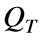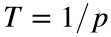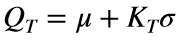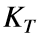# Problem 57620. Compute frequency factors for the normal distribution

In frequency analysis in hydrology, the streamflowcorresponding to a specified exceedance probability p (or return period) can be computed aswhere μ and σ are the mean and standard deviation of the streamflow series, respectively, andis the frequency factor.
Write a function to compute the frequency factor for the normal distribution given the exceedance probability as a vector.

### Solution Stats

22.22% Correct | 77.78% Incorrect
Last Solution submitted on Jun 01, 2023

### Community Treasure Hunt

Find the treasures in MATLAB Central and discover how the community can help you!

Start Hunting!